# Rhombus

For other uses, see Rhombus (disambiguation).
RhombusTwo rhombi.
Edges and vertices 4
Schläfli symbol { } + { }
Coxeter diagramSymmetry group Dihedral (D2), , (*22), order 4
Area(half the product of the diagonals)
Dual polygon rectangle
Properties convex, isotoxal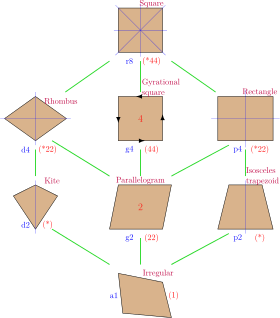The rhombus has a square as a special case, and is a special case of a kite and parallelogram.

In Euclidean geometry, a rhombus(◊) (plural rhombi or rhombuses) is a simple (non-self-intersecting) quadrilateral whose four sides all have the same length. Another name is equilateral quadrilateral, since equilateral means that all of its sides are equal in length. The rhombus is often called a diamond, after the diamonds suit in playing cards which resembles the projection of an octahedral diamond, or a lozenge, though the former sometimes refers specifically to a rhombus with a 60° angle (see Polyiamond), and the latter sometimes refers specifically to a rhombus with a 45° angle.

Every rhombus is a parallelogram and a kite. A rhombus with right angles is a square.

## Etymology

The word "rhombus" comes from Greek ῥόμβος (rhombos), meaning something that spins, which derives from the verb ρέμβω (rhembō), meaning "to turn round and round". The word was used both by Euclid and Archimedes, who used the term "solid rhombus" for two right circular cones sharing a common base.

The surface we refer to as rhombus today is a cross section of this solid rhombus through the apex of each of the two cones.

## Characterizations

A simple (non-self-intersecting) quadrilateral is a rhombus if and only if it is any one of the following:

• a quadrilateral with four sides of equal length (by definition)
• a quadrilateral in which the diagonals are perpendicular and bisect each other
• a quadrilateral in which each diagonal bisects two opposite interior angles
• a quadrilateral ABCD possessing a point O in its plane such that the four triangles ABO, BCO, CDO, and DAO are all congruent
• a parallelogram in which a diagonal bisects an interior angle
• a parallelogram in which at least two consecutive sides are equal in length
• a parallelogram in which the diagonals are perpendicular (an orthodiagonal parallelogram)

## Equation

The sides of a rhombus centered at the origin, with diagonals each falling on an axis, consist of all points (x, y) satisfyingThe vertices are atandThis is a special case of the superellipse, with exponent 1.

## Basic properties

Every rhombus has two diagonals connecting pairs of opposite vertices, and two pairs of parallel sides. Using congruent triangles, one can prove that the rhombus is symmetric across each of these diagonals. It follows that any rhombus has the following properties:

The first property implies that every rhombus is a parallelogram. A rhombus therefore has all of the properties of a parallelogram: for example, opposite sides are parallel; adjacent angles are supplementary; the two diagonals bisect one another; any line through the midpoint bisects the area; and the sum of the squares of the sides equals the sum of the squares of the diagonals (the parallelogram law). Thus denoting the common side as a and the diagonals as p and q, in every rhombusNot every parallelogram is a rhombus, though any parallelogram with perpendicular diagonals (the second property) is a rhombus. In general, any quadrilateral with perpendicular diagonals, one of which is a line of symmetry, is a kite. Every rhombus is a kite, and any quadrilateral that is both a kite and parallelogram is a rhombus.

A rhombus is a tangential quadrilateral. That is, it has an inscribed circle that is tangent to all four sides.

## Area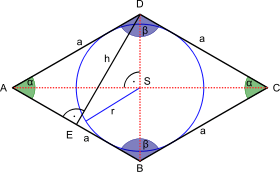A rhombus. Each angle marked with a black dot is a right angle. The height h is the perpendicular distance between any two non-adjacent sides, which equals the diameter of the circle inscribed. The diagonals of lengths p and q are the red dotted line segments.

As for all parallelograms, the area K of a rhombus is the product of its base and its height (h). The base is simply any side length a:The area can also be expressed as the base squared times the sine of any angle:or in terms of the height and a vertex angle:or as half the product of the diagonals p, q:or as the semiperimeter times the radius of the circle inscribed in the rhombus (inradius):Another way, in common with parallelograms, is to consider two adjacent sides as vectors, forming a bivector, so the area is the magnitude of the bivector (the magnitude of the vector product of the two vectors), which is the determinant of the two vectors' Cartesian coordinates: K = x1y2x2y1.

The inradius (the radius of a circle inscribed in the rhombus), denoted by r, can be expressed in terms of the diagonals p and q as:## Dual properties

The dual polygon of a rhombus is a rectangle:

• A rhombus has all sides equal, while a rectangle has all angles equal.
• A rhombus has opposite angles equal, while a rectangle has opposite sides equal.
• A rhombus has an inscribed circle, while a rectangle has a circumcircle.
• A rhombus has an axis of symmetry through each pair of opposite vertex angles, while a rectangle has an axis of symmetry through each pair of opposite sides.
• The diagonals of a rhombus intersect at equal angles, while the diagonals of a rectangle are equal in length.
• The figure formed by joining the midpoints of the sides of a rhombus is a rectangle and vice versa.

## Other properties

As topological square tilings As 30-60 degree rhombille tiling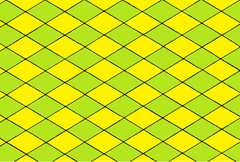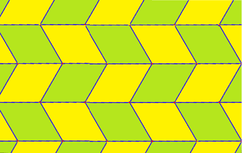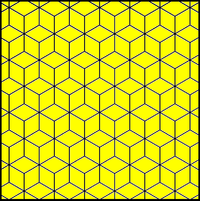Some polyhedra with all rhombic faces
Identical rhombi Two types of rhombi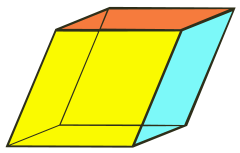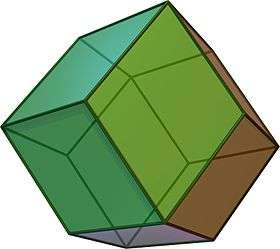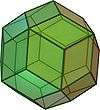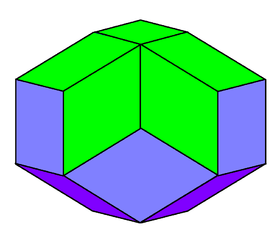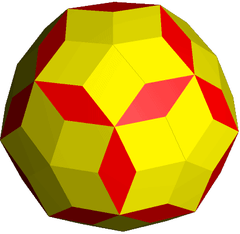Rhombohedron Rhombic dodecahedron Rhombic triacontahedron Rhombic icosahedron Rhombic enneacontahedron

## As a Varignon parallelogram

The Varignon parallelogram of an equidiagonal quadrilateral is a rhombus.

## As the faces of a polyhedron

A rhombohedron is a three-dimensional figure like a cube, except that its six faces are rhombi instead of squares.

The rhombic dodecahedron is a convex polyhedron with 12 congruent rhombi as its faces.

The rhombic triacontahedron is a convex polyhedron with 30 golden rhombi (rhombi whose diagonals are in the golden ratio) as its faces.

The great rhombic triacontahedron is a nonconvex isohedral, isotoxal polyhedron with 30 intersecting rhombic faces.

The rhombic hexecontahedron is a stellation of the rhombic triacontahedron. It is nonconvex with 60 golden rhombic faces with icosahedral symmetry.

The rhombic enneacontahedron is a polyhedron composed of 90 rhombic faces, with three, five, or six rhombi meeting at each vertex. It has 60 broad rhombi and 30 slim ones.

The trapezo-rhombic dodecahedron is a convex polyhedron with 6 rhombic and 6 trapezoidal faces.

The rhombic icosahedron is a polyhedron composed of 20 rhombic faces, of which three, four, or five meet at each vertex. It has 10 faces on the polar axis with 10 faces following the equator.

## References

1. Note: Euclid's original definition and some English dictionaries' definition of rhombus excludes squares, but modern mathematicians prefer the inclusive definition.
2. inclusive usage
3. ῥόμβος, Henry George Liddell, Robert Scott, A Greek-English Lexicon, on Perseus
4. ρέμβω, Henry George Liddell, Robert Scott, A Greek-English Lexicon, on Perseus
5. The Origin of Rhombus
6. Zalman Usiskin and Jennifer Griffin, "The Classification of Quadrilaterals. A Study of Definition", Information Age Publishing, 2008, pp. 55-56.
7. Owen Byer, Felix Lazebnik and Deirdre Smeltzer, Methods for Euclidean Geometry, Mathematical Association of America, 2010, p. 53.
8. Paris Pamfilos, "A Characterization of the Rhombus", Forum Geometricorum 16, 2016, 331–336. http://forumgeom.fau.edu/FG2016volume16/FG201640.pdf
9. WildLinAlg episode 4, Norman J Wildberger, Univ. of New South Wales, 2010, lecture via youtube
10. de Villiers, Michael, "Equiangular cyclic and equilateral circumscribed polygons", Mathematical Gazette 95, March 2011, 102-107.
11. de Villiers, Michael (2009), Some Adventures in Euclidean Geometry, Dynamic Mathematics Learning, p. 58, ISBN 9780557102952.Look up rhombus in Wiktionary, the free dictionary.Wikimedia Commons has media related to Rhombus.